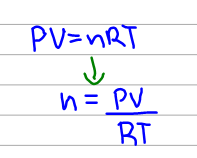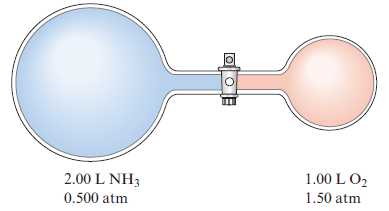# Problem: Nitric acid is produced commercially by the Ostwald process. In the first step ammonia is oxidized to nitric oxide:4NH3(g) + 5O2(g) → 4NO(g) + 6H2O(g)Assume this reaction is carried out in the apparatus diagramed below.The stopcock between the two reaction containers is opened, and the reaction proceeds using proper catalysts. Calculate the partial pressure of NO after the reaction is complete. Assume 100% yield for the reaction, assume the final container volume is 3.00 L, and assume the temperature is constant.

###### FREE Expert Solution

We are given the initial partial pressures and volumes of the reactants, we have to use the ideal gas law to calculate the moles of each. The ideal gas law can be manipulated to isolate the moles :Now before we calculate, make sure that the units are the same as the units of the gas constant R. If not, we convert. Assume room temperature.

91% (455 ratings)###### Problem Details

Nitric acid is produced commercially by the Ostwald process. In the first step ammonia is oxidized to nitric oxide:

4NH3(g) + 5O2(g) → 4NO(g) + 6H2O(g)

Assume this reaction is carried out in the apparatus diagramed below.The stopcock between the two reaction containers is opened, and the reaction proceeds using proper catalysts. Calculate the partial pressure of NO after the reaction is complete. Assume 100% yield for the reaction, assume the final container volume is 3.00 L, and assume the temperature is constant.